# Multiples of 9

Multiples of 9• Multiples of 9 are numbers that can be divided exactly by 9.
• The first few multiples of 9 are the numbers in the 9 times table.
• For example 3 × 9 = 27 and so, the third multiple of nine is 27.
• For example 100 × 9 = 900 and so, the hundredth multiple of 9 is 900.
• The first multiples of 9 are 9, 18, 27, 36, 45, 54, 63, 72, 81, 90, 99 and 108.

A multiple of 9 is any number that can be divided by 9 exactly, leaving no remainder.#### Multiples of 9 Flashcards

Click on the multiples of 9 flashcards below to memorise the 9 times table:

×
×

=#### Multiples of 9: Interactive Questions

Multiples of 9: Interactive Questions

# Multiples of 9

## What are the Multiples of 9?

The multiples of 9 are any numbers that can be divided exactly by 9 to leave no remainder. The first ten multiples of 9 are 9, 18, 27, 36, 45, 54, 63, 72, 81 and 90. We can continue to find more multiples of 9 by adding on 9 each time. There are an infinite number of multiples of 9.

Here is the 9 times table, which makes up the first 12 multiples of 9: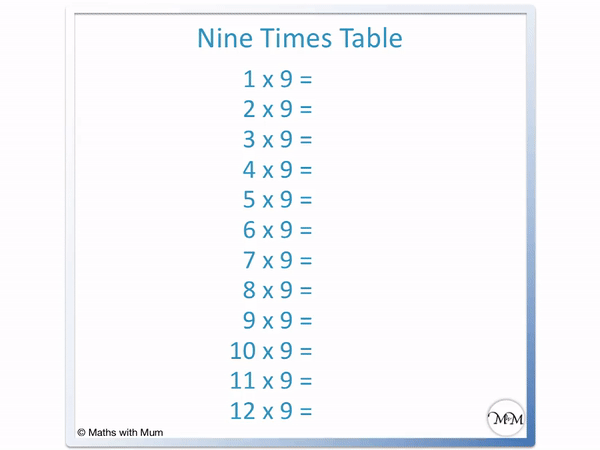The multiples of 9 all have a difference of 9 between them. Add 9 from one multiple of 9 to get to the next multiple of 9.## Patterns in the Multiples of 9

The multiples of 9 all have digits that add up to another multiple of 9. There is also a diagonal pattern created by shading the multiples of 9 on a number grid.

Here are the multiples of 9 shown shaded on a number grid.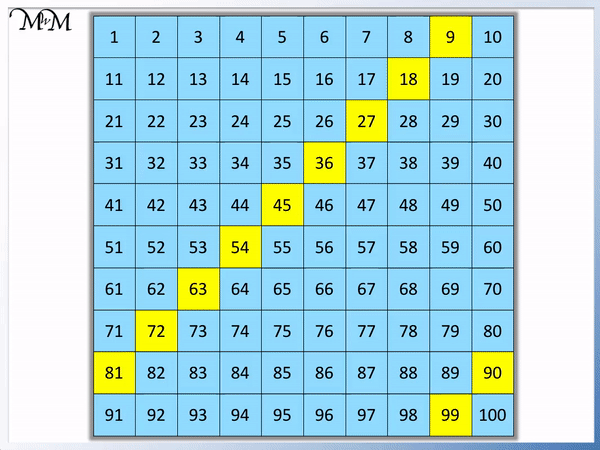We can see that there is a clear diagonal pattern shown in the multiples of 9. This pattern can help us figure out the next multiple of 9.

When teaching the multiples of 9, it can be helpful to show a number grid, where the diagonal pattern is shown.

We can see further patterns in the multiples of 9 by reading their digits.

The digit at the end of the number decreases by 1 as we move from one multiple of 9 to the next. For example, 9 ends in 9, 18 ends in 8 and 27 ends in 7.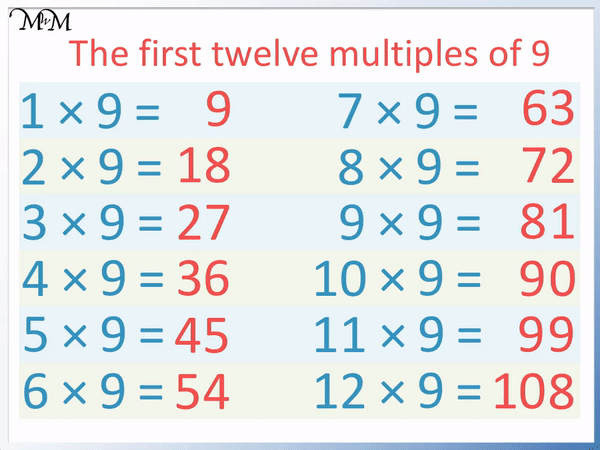Another pattern is that the number in the tens column decreases by 1 as we move from one multiple of 9 to the next. For example, the tens digit in 9 is 0, in 18 it is 1, in 27 it is 2 and so on.

An interesting pattern in the multiples of 9 is that the sum of the digits add to a multiple of 9. The first 10 multiples of 9 have digits that always sum to make 9, which can make them easier to memorise.

For example, when looking at the numbers of 9, 18, 27 and 36, we have 9 = 9, 1 + 8 = 9, 2 + 7 = 9 and 3 + 6 = 9. This pattern continues all the way up to 90, which has 9 + 0 = 9.

With larger multiples of 9, there is a pattern of the digits adding to any multiple of 9.

For example, with 12 × 9 = 108, we can see that the sum of the digits adds to 9. 1 + 0 + 8 = 9.The sum of the digits add to 9 or any other multiple of 9.Here is the example of 21 × 9 = 189.Here we can see that 1 + 8 + 9 = 18. Again the sum of the digits of this multiple of 9 make another multiple of 9. 18 is 2 × 9.## How to Identify Multiples of 9

All multiples of 9 must have digits that sum to make a smaller multiple of 9. For example, 5976 is a multiple of 9 because 5 + 9 + 7 + 6 = 27 and 27 is 3 × 9. 3299 is not a multiple of 9 because 3 + 2 + 9 + 9 = 23, which is not a multiple of 9.

To decide if a number is a multiple of 9, follow these steps:

1. Add the digits of the number.
2. If the result is a multiple of 9, then the original number is a multiple of 9.
3. If the result is not a multiple of 9, then the original number is not a multiple of 9.
4. If you are not sure if the result is a multiple of 9, add the digits of this number and use the above steps to decide.

For example, here is 5976.

The first step is to add the digits.

5 + 9 + 7 + 6 = 27.

27 is a multiple of 9 because 3 × 9 = 27.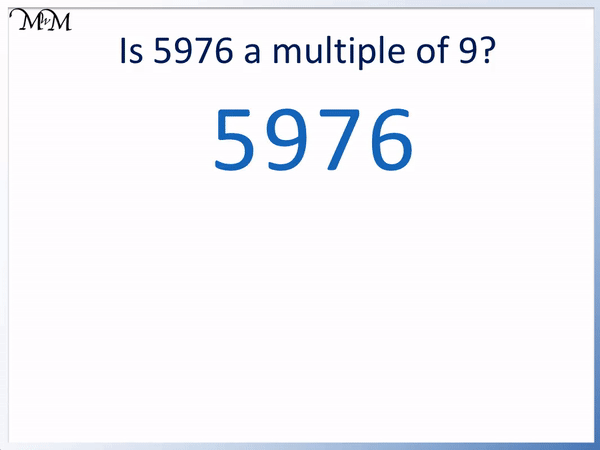If you were unsure if 27 was a multiple of 9, then you can add the digits of this number. 2 + 7 = 9 and so, 27 is a multiple of 9.

Because the sum of the digits of 5976 is a multiple of 9, we know that 5976 is also a multiple of 9.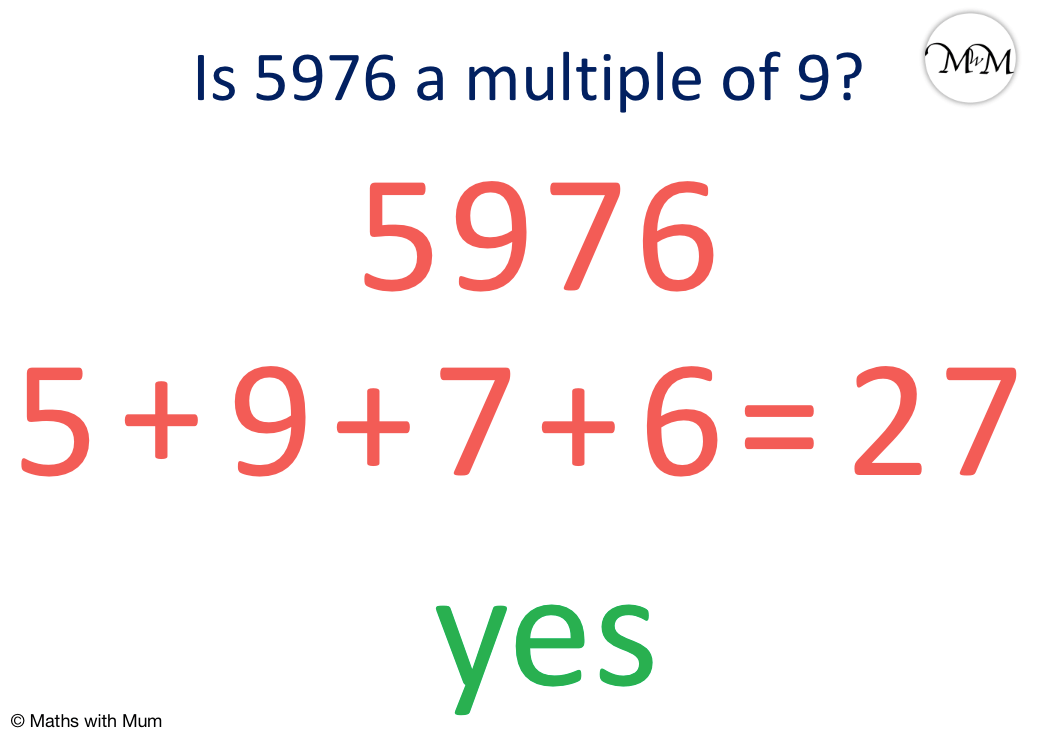Here is an example of a number that is not a multiple of 9. We will use the rule to show that 3299 is not a multiple of 9.

The first step is to add the digits.

3 + 2 + 9 + 9 = 23.

23 is not a multiple of 9 and so, 3299 is not a multiple of 9.

We know that 23 is not a multiple of 9 because 2 + 3 = 5, which is not a number in the 9 times table.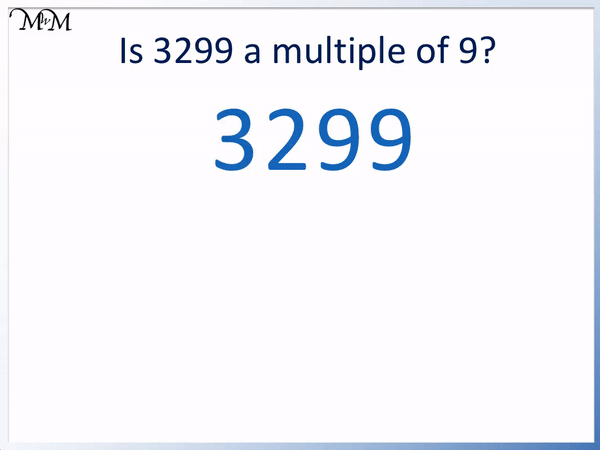The rule for identifying multiples of 9 works for all numbers, no matter how large.

Here is another example, testing if the number 7635 is a multiple of 9 or not.7 + 6 + 3 + 5 = 21. 21 is not a multiple of 9 and so 7635 is not a multiple of 9. We know that 21 is not a multiple of 9 because 2 + 1 = 3, which is not in the 9 times table.

## Is Zero a Multiple of 9?

Zero is a multiple of 9 because it can be divided exactly by 9 to leave no remainder. 0 ÷ 9 = 0. We can also see that zero is a multiple of 9 because 0 × 9 = 0. Zero is a multiple of every number.

## What are the Multiples of 9 up to 100?

There are 11 multiples of 9 that are smaller than 100. They are:

9, 18, 27, 36, 45, 54, 63, 72, 81, 90 and 99.## Multiples of 9 List

Here is a list of multiples of 9:

9, 18, 27, 36, 45, 54, 63, 72, 81, 90, 99, 108, 117, 126, 135, 144, 153, 162, 171, 180, 189, 198, 207, 216, 225, 234, 243, 252, 261, 270, 279, 288, 297, 306, 315, 324, 333, 342, 351, 360, 369, 378, 387, 396, 405, 414, 423, 432, 441, 450, 459, 468, 477, 486, 495, 504, 513, 522, 531, 540, 549, 558, 567, 576, 585, 594, 603, 612, 621, 630, 639, 648, 657, 666, 675, 684, 693, 702, 711, 720, 729, 738, 747, 756, 765, 774, 783, 792, 801, 810, 819, 828, 837, 846, 855, 864, 873, 882, 891, 900, 909, 918, 927, 936, 945, 954, 963, 972, 981, 990 and 999.

There are 111 multiples of 9 up to 1000.Now try our lesson on Finding Factors of a Number where we learn how to find factors.error: Content is protected !!